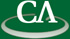## Random Polynomial Dilations

### Michael Monagan, CECM, Simon Fraser Univeristy

Friday January 28th, 12:30pm, online.

Abstract:

Given a polynomial f(x, y, z) over a field K and non-zero point a, b, c in K3, the polynomial f(ax, by, cz) is called a dilation of f.   This mapping is invertible, and importantly, unlike the shift f(x+a, y+b, z+c), it preserves the sparsity of f.   Our idea is to pick a, b, c at random from a large subset S of K and use the random dilation f(ax, by, cz) to avoid problem evaluation points in algorithms that use sparse polynomial interpolation. In particular we show how it can be used to avoid "unlucky evaluation points" in the modular GCD algorithm. In previous work, Giesbrecht and Roche used a random dilation to randomize the coefficients of f.